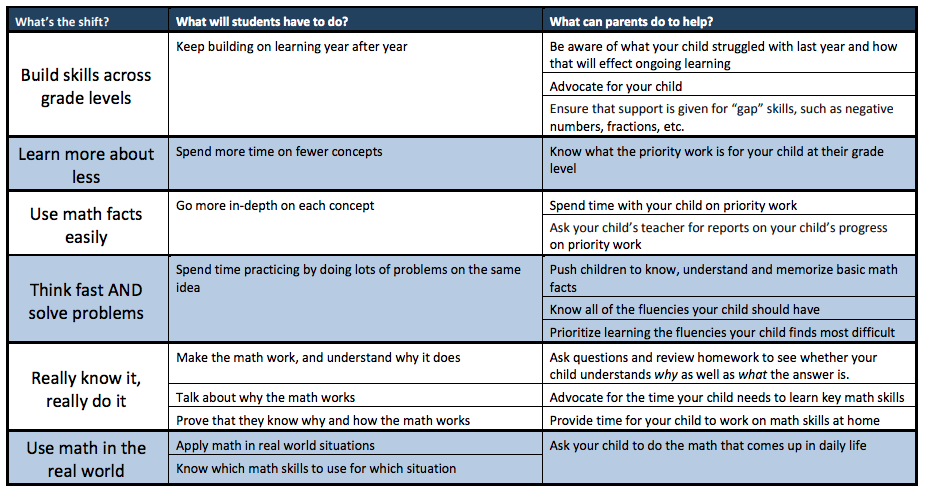# Culver City Unified School District# Math

 What are the Common Core Mathematics Standards?

The Common Core Standards reflect the knowledge and skills that our students need in order to be successful in college and careers.  The standards require that students gain knowledge through reading, writing, speaking, and listening.  California is one of 45 states that have voluntarily adopted the Common Core State Standards.  These Common Core State Standards  were designed to prepare students for the rigor and performance expectations of college and the workplace.

 What are the Performance Expectations (Standards) for each grade?

 How is Math different than when I went to school?

1) Greater focus on fewer topics
The Common Core calls for greater focus in mathematics.  Rather than racing to cover many topics in a mile-wide, inch-deep curriculum, the standards ask math teachers to significantly narrow and deepen the time and energy that are spent in the classroom.
• In grades K-2: Concepts, skills, and problem solving related to addition and subtraction.
• In grades 3-5: Concepts, skills, and problem solving related to multiplication and division of whole numbers and fractions
• In grade 6: Ratios and proportional relationships, and early algebraic expressions and equations.
• In grade 7:  Ratios and proportional relationships, and arithmetic of rational numbers
• In grade 8: Linear algebra and linear functions

This focus helps students gain strong foundations, including a solid understanding of concepts, a high degree of procedural skill and fluency, and the ability to apply the math they know to solve problems inside and outside of the classroom.

Mathematics is not a list of disconnected topics, tricks, or mnemonics; it is a coherent body of knowledge made up of interconnected concepts.  For example, in 4th grade, students must "apply and extend previous understanding of multiplication to multiply a fraction by a whole number" (Standard 4.NF.4).  This extends to 5th grade, when students are expected to build on that skill to "apply and extend previous understanding of multiplication to multiply a fraction or whole number by a fraction" (Standard 5.N.F.4).  Each standard is not a new event, but an extension of previous learning.

3) Rigor
Pursue conceptual understanding, procedural skills and fluency, and application with equal intensity
Rigor refers to deep, authentic command of mathematical concepts, not making math harder or introducing topics at earlier grades.  To help students meet the standard, educators will need to pursue conceptual understanding (see math as more than a set of mnemonics or discrete procedures), Procedural skill and fluency (speed and accuracy in calculation), and application (use math situations that require math knowledge)

4) Use math in the real world

 How can I support my Child in Math?

Expectations for Students and Ideas for ParentsThe Council of the Great City Schools has developed content and grade-specific parent roadmaps that provide detailed information for parents about the expectations of the Common Core Mathematics.  These roadmaps include examples of grade-level focus.Family Math Roadmaps: Kindergarten 1st Grade 2nd Grade 3rd Grade 4th Grade 5th Grade 6th Grade 7th Grade 8th Grade HS

Other Math Resources:
 Math Acceleration Parent Information Meeting (4th grade parents/guardians)

The following recording will review the the K-12 math pathway and explain the difference between state recommended math, accelerated math, and double accelerated math pathway.

 Mathlete

What is Mathletes?

A Mathlete is a person who competes in mathematic competitions at elementary and middle school.  More specifically, a Mathelete is a student who participates in any of the MATHCOUNTS program.

How can you prepare?

Learning Mathematics Through Games:  Series of web pages discussing rationale for and different types of games, then suggestion of specific games how to make your own.

Mathematical Olympiads for Elementary and Middle School (MOEMS)